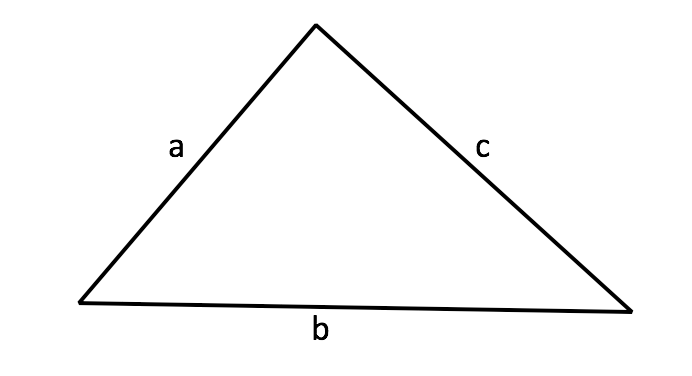# Triangle Perimeter Calculator

## Perimeter=a+b+c

Side A: Base Side B:The triangle is one of the most useful and common shapes in all of geometry. Triangles are the simplest two-dimensional shapes that you can create.

Triangles, which are typically made up of 3 sides and 3 angles, can be classified by their properties; namely, their vertices, the size of each angle, and the type of angles.

There are three different types of triangles based on their angles; right triangle, obtuse triangle, and acute triangle. There are three different types of triangles based on their sides; scalene, isosceles, or equilateral.

Triangles are enclosed shapes with three sides. The perimeter of the shape is the distance around the outside edge, which is usually calculated by adding up the lengths of each side. To find the perimeter of a triangle, simply add together the lengths of all three sides.

The triangle's perimeter is found by adding together a's length, b's length, and c's length.